﻿ 岸电电缆绝缘气隙热源等效模型及载流量的影响研究

# 岸电电缆绝缘气隙热源等效模型及载流量的影响研究Study on the Influence of Insulation Air Gap Defects on the Current Carrying Capacity of Shore Power Cable

Abstract: The special use condition of the shore power cable is likely to cause cracking air gap in cable insulation then cause air gap discharge. Continuous air gap discharge will cause local temperature to rise, which will affect the current carrying capacity of shore power cable. In this paper, the multi-physics coupling analysis method is used to establish the air gap defect model of shore power cable. The influence of different air gap discharge power on the temperature distribution of the shore power cable is studied, and the current carrying capacity of the shore power cable under different air gap discharge power is calculated. The results show that the temperature distribution of the cable is quadratic with the load current and linear with the air gap power. With the increase of air gap discharge power, the defect phase ampacity decreases rapidly, while the non-defect phase ampacity decreases slowly and then sharply. As the air gap discharge power increases, the rated current of the defect phase decreases rapidly, the non-defect phase drops slowly and then drops rapidly. The research results of this paper can provide reference for the defect detection of shore power cable and the control of load current.

1. 引言

2. 岸电电缆气隙缺陷模型

2.1. 物理模型

2.2. 数学模型

1) 温度场计算模型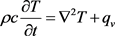(1)

2) 电缆本体热损耗计算

$\nabla ×\frac{1}{{\mu }_{0}{\mu }_{r}}\nabla ×\stackrel{˙}{A}=\left(\sigma +j\omega {\epsilon }_{0}{\epsilon }_{r}\right)\left(-j\omega \stackrel{˙}{A}-\nabla \stackrel{˙}{\phi }\right)$ (2)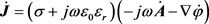(3)

$\nabla \cdot \stackrel{˙}{A}+jw{\mu }_{0}{\mu }_{r}{\epsilon }_{0}{\epsilon }_{r}\stackrel{˙}{\phi }=0$ (4)

${q}_{1}=\frac{1}{2}{\int }_{V}\frac{\stackrel{˙}{J}\cdot {\stackrel{˙}{J}}^{*}}{\gamma }\text{d}V$ (5)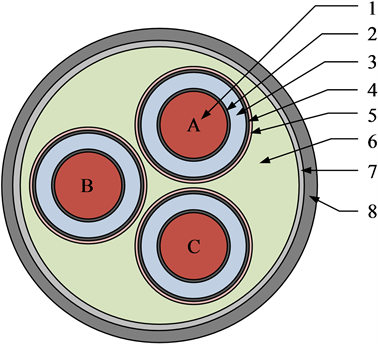1导体(CO)；2导体屏蔽(CS)；3绝缘体(IN)；4绝缘屏蔽(IS)； 5金属屏蔽(MS)；6填充体(FI)；7内护套(IS)；8外护套(OS)

Figure 1. 10 kV three-core shore cable structure diagram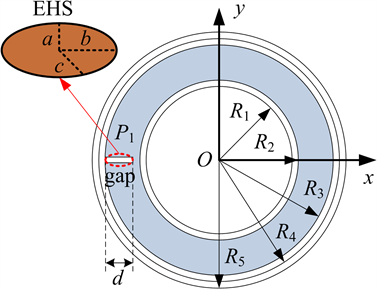Figure 2. Shoreline cable air gap modelTable 1. Shore cable material properties

$\gamma =\frac{{\gamma }_{ref}}{1+\beta \left(T-{T}_{ref}\right)}$ (6)

3) 气隙损耗计算

${q}_{e}\left(x,y,z\right)={q}_{em}\mathrm{exp}\left(-\frac{3{x}^{2}}{{a}^{2}}-\frac{3{y}^{2}}{{b}^{2}}-\frac{3{z}^{2}}{{c}^{2}}\right)$ (7)

${P}_{2}=8{\int }_{0}^{\infty }{\int }_{0}^{\infty }{\int }_{0}^{\infty }{q}_{e}\left(x,y,z\right)\text{d}x\text{d}y\text{d}z=$ ${q}_{em}\frac{abc\pi \sqrt{\pi }}{3\sqrt{3}}$ (8)

${q}_{em}=\frac{3\sqrt{3}{P}_{2}}{\pi \sqrt{\pi }abc}$ (9)

${P}_{2}={U}_{g}{I}_{g}$ (10)

${q}_{v}={q}_{1}+{q}_{2}$ (11)

2.3. 边界条件

${A|}_{S}=0$ (12)

${-\lambda \left(\frac{\partial T}{\partial n}\right)|}_{S}=h\left({T}_{S}-{T}_{f}\right)$ (13)

${-\lambda \left(\frac{\partial T}{\partial n}\right)|}_{S}=\xi b\left({T}_{S}^{4}-{T}_{f}^{4}\right)$ (14)

3. 结果分析

3.1. 岸电电缆气隙放电下的温度分布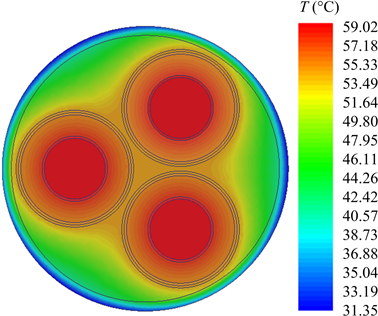Figure 3. Normal cable temperature distribution cloud map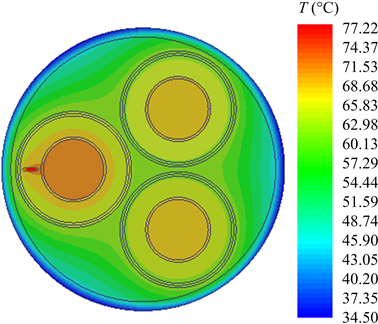Figure 4. Defect cable temperature distribution cloud map

3.2. 气隙放电对岸电电缆载流量的影响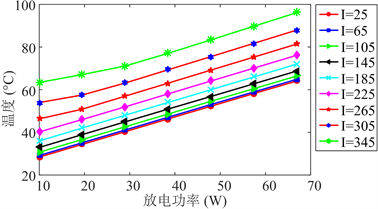Figure 5. B phase temperature distribution curve of shore power cable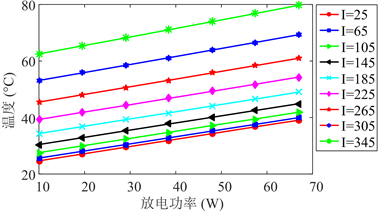Figure 6. Shoreline cable A phase temperature distribution curve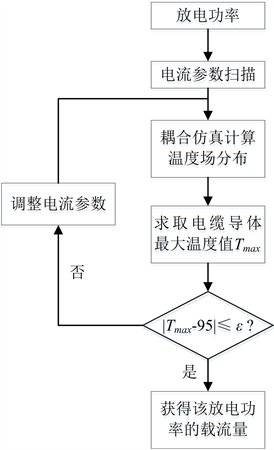Figure 7. Flow chart calculation flowchart

${T}_{1}\left({I}_{d}\right)={c}_{1}{I}_{d}^{2}+{c}_{2}{I}_{d}+{c}_{3}$ (15)

${T}_{b}\left({P}_{2}\right)={k}_{1}{P}_{2}+{T}_{1}\left({I}_{d}\right)$ (16)

${T}_{a}\left({P}_{2}\right)={k}_{2}{P}_{2}+{T}_{1}\left({I}_{d}\right)$ (17)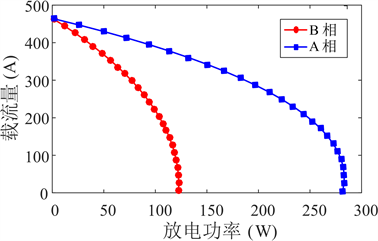Figure 8. Current carrying capacity of different air gap discharge power

4. 结论

1) 缺陷相温度比非缺陷相温度高，但是其温度变化率相同。

2) 分别对非缺陷电缆和缺陷电缆进行耦合仿真，发现电缆温度分布与负荷电流呈二次函数关系，与气隙功率呈线性关系。

3) 随着气隙放电功率增加，缺陷相载流量迅速下降，而非缺陷相载流量下降先缓后急。这对其中一相发生故障后，另两相继续工作的负荷控制具有重要意义。

 Burgel, A.P. (2007) Air Pollution from Ships: Recent Developments. WMU Journal of Maritime Affairs, 6, 217-224.
https://doi.org/10.1007/BF03195116

 Cuculic, A., Vucetic, D. and Tomas, V. (2011) High Voltage Shore Connection. 53rd International Symposium ELMAR, Zadar, 14-16 September 2011, 257-259.

 Okubo, H., Hayakawa, N. and Matsushita, A. (2002) The Relationship between Partial Discharge Current Pulse Waveforms and Physical Mechanisms. IEEE Electrical Insulation Magazine, 18, 38-45.
https://doi.org/10.1109/MEI.2002.1014966

 Xu, X., Bengtsson, T., Hammarstrom, T., Blennow, J. and Gubanski, S.M. (2015) Loss Current Studies of Partial Discharge Activity. IEEE Transactions on Dielectrics and Electrical Insulation, 22, 472-481.
https://doi.org/10.1109/TDEI.2014.004581

 杨帆, 杨旗, 程鹏, 等. 电缆接头内部气隙放电缺陷下的绝缘劣化程度表征方法[J]. 电工技术学报, 2017, 32(2): 24-32.

 刘庆明, 汪建平, 李磊, 张云明, 宇灿. 电火花放电能量及其损耗的计算[J]. 高电压技术, 2014, 40(4): 1255-1260.

 Alisoy, H.Z., Yeroglu, C., Koseoglu, M. and Hansu, F. (2005) Investigation of the Characteristics of Dielectric Barrier Discharge in the Transition Region. Journal of Physics D: Applied Physics, 38, 4272-4277.
https://doi.org/10.1088/0022-3727/38/24/002

 Liu, K., Yang, F., Wang, S., Gao, B. and Xu, C. (2018) The Research on the Heat Source Characteristics and the Equivalent Heat Source of the Arc in Gaps. International Journal of Heat and Mass Transfer, 124, 177-189.
https://doi.org/10.1016/j.ijheatmasstransfer.2018.03.048

 郝艳捧, 涂恩来, 阳林, 戴栋. 基于气隙伏安特性研究大气压氦气辉光放电的模式和机理[J]. 电工技术学报, 2010, 25(7): 24-30.

 付洋洋, 罗海云, 邹晓兵, 王强, 王新新. 棒-板电极下缩比气隙辉光放电相似性的仿真研究[J]. 物理学报, 2014, 63(9): 095206.

 潘俊, 方志. 多脉冲均匀介质阻挡放电特性的仿真及实验研究[J]. 高电压技术, 2012, 38(5): 1132-1140.

 刘晓妍. 电力电缆载流量计算方法的研究[J]. 电子测试, 2017(8): 35-36.

 郝艳捧, 陈云, 阳林, 等. 高压直流海底电缆电-热-流多物理场耦合仿真[J]. 高电压技术, 2017(11): 68-76.

 Del Pino Lopez, J.C. and Cruz Romero, P. (2011) Influence of Different Types of Magnetic Shields on the Thermal Behavior and Ampacity of Underground Power Cables. IEEE Transactions on Power Delivery, 26, 2659-2667.
https://doi.org/10.1109/TPWRD.2011.2158593

Top